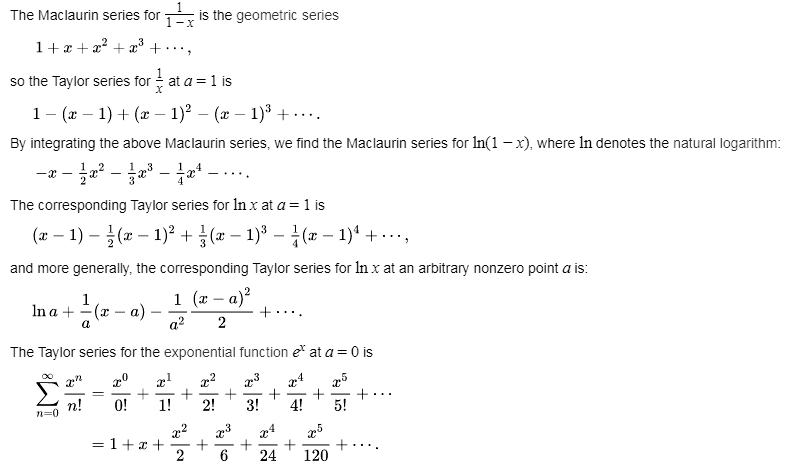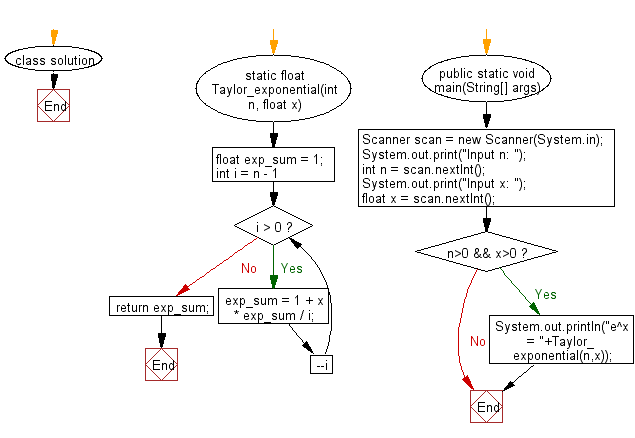﻿ Java Math Exercises: Calculate e raise to the power x using sum of first n terms of Taylor Series - w3resource# Java Math Exercises: Calculate e raise to the power x using sum of first n terms of Taylor Series

## Java Math Exercises: Exercise-25 with Solution

From Wikipedia,
In mathematics, a Taylor series is a representation of a function as an infinite sum of terms that are calculated from the values of the function's derivatives at a single point.
Example:
The Taylor series for any polynomial is the polynomial itself.The above expansion holds because the derivative of ex with respect to x is also ex, and e0 equals 1.
This leaves the terms (x − 0)n in the numerator and n! in the denominator for each term in the infinite sum.
Write a Java program to calculate e raise to the power x using sum of first n terms of Taylor Series.

Sample Solution:

Java Code:

``````import java.util.*;

class solution {

static float Taylor_exponential(int n, float x)
{
float exp_sum = 1;

for (int i = n - 1; i > 0; --i )
exp_sum = 1 + x * exp_sum / i;

return exp_sum;
}

public static void main(String[] args)
{
Scanner scan = new Scanner(System.in);
System.out.print("Input n: ");
int n = scan.nextInt();
System.out.print("Input x: ");
float x = scan.nextInt();
if (n>0 && x>0)
{
System.out.println("e^x = "+Taylor_exponential(n,x));
}
}
}
``````

Sample Output:

```Input n:  25
Input x:  5
e^x = 148.41316
```

Flowchart:Java Code Editor:

What is the difficulty level of this exercise?

﻿

## Java: Tips of the Day

Parsing dates:

```import java.io.*;
import java.util.*;
import java.text.*;

String s = "2001/09/23 14:39";

SimpleDateFormat formatter = new SimpleDateFormat ("yyyy/MM/dd H:mm");
Date d = formatter.parse(s, new ParsePosition(0));
```